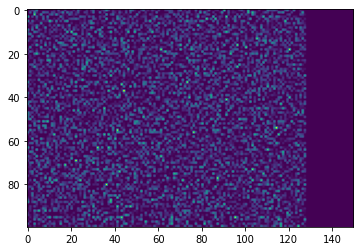# About a fifth of the gradients are lost if using .cuda() not inplace

I think I found a pytorch bug but I’m not entirely certain.
Before I’m writing an issue I’ll post it here.

I have uploaded the sample code to google colab and the output with the error is visible in the output field. colab link here https://colab.research.google.com/drive/1UC7mL2FS4Gz5HIQuSeQaSnWFynQmIHzn#scrollTo=V4MjwHAljuTN&uniqifier=2
Dont forget to activate the gpu in notebook settings.

I am generating 4 RGB random images and put them to my gpu.

``````images = torch.rand(1,3,4,widht,height)
images = images.cuda() # this causes the error
``````

after that I feed the images into a Maxpool3d and use a dummy loss so I can calculate
the gradients of my input images.

``````x = nn.MaxPool3d(kernel_size=(1, 2, 2), stride=(1, 2, 2), padding=(0, 0, 0))(images)
criterion = nn.MSELoss()
label = torch.ones_like(x)
loss = criterion(x, label)
loss.backward()
``````

If I now analyze the gradients of the images

`````` grads_images = images.grad
``````

I noticed that about a third of the gradients are very close to zero although there is no
reason for that.

To make this problem clear I am visualizing the gradients.
I take only one image, use the absolute and scale them between 0 and 1. Then I add
the gradients of the red, green and blue channel together so i get a
numpy array with size [widht, height]

The values in this array can now be visualized with a colormap of plt.You can see the right fifth of the gradients are ~0

But If I change one line

`````` images = torch.rand(1,3,4,widht,height)
images.cuda() # this fixes the gradient error
``````

The gradients are no longer ~0 in the right fifht of the image.
(I’m a new user so I can’t paste more than one image so the new image is here https://imgur.com/a/ruuQQr4 and also in the colab)

If you don’t want to use colab, the full code is here:

``````import torch
import torch.nn as nn
import matplotlib.pyplot as plt
import numpy as np
%matplotlib inline
"""
I create 4 random images with batchsize 1 and channelsize 3 (rgb)
Then I use a 3d Maxpool
Then I want to visualize the gradients of our images with a target image
that consists of only ones.

The gradients dissapear if I overwrite my input images with images.cuda()
"""
height = 100 # width of our image
width = 150 # height of our image
images = torch.rand(1,3,4,height,width) # create 4 images with this height and width
images = images.cuda() # This creates the error
"""IF YOU REPLACE THE ABOVE LINE WITH images.cuda() IT WORKS AS INTENDED"""

pooled_imgs = nn.MaxPool3d(kernel_size=(1, 2, 2), stride=(1, 2, 2), padding=(0, 0, 0))(images)

# create a dummy target (same size as output with ones) and calculate the mse
criterion = nn.MSELoss()
label = torch.ones_like(pooled_imgs)
loss = criterion(pooled_imgs, label)
loss.backward()

# scale gradients between 0 and 1

# scaled grads has shape [Batchsize, Channels(RGB),amnt_images, width, height]
grad_pic = scaled_grads[0, :,0, :, :] # use batch 0 and the first image in our depth
# grad pic has no shape [3,height,width]

grad_pic = np.transpose(grad_pic, (1, 2, 0)) # shape now [height, width, 3]
# I want to add all gradients of the 3 channels (red, green blue)
# so I can use a plt colormap

``````
2 Likes

Hi,

Running with a brand new env there, I get the proper result for each cell though. Can you try again on your side?

Also `image.cuda()` does nothing! You can remove this line and it will have the exact same behavior. (.cuda() cannot be done inplace).

It seems that colab switched to torch 1.6 at the time between my question and your reply.
At first glance I am also no longer able to reproduce the error with torch 1.6 in the colab.

I am still able to reproduce the bug with torch 1.4 with copy pasting the first cell to my local machine (deleting %matplotlib inline and adding as last line plt.show())
I don’t have torch 1.5 installed on my local machine but the code worked yesterday in google colab which was using torch 1.5.

I can’t use

!pip install torch==1.5.0 torchvision==0.6.0

in colab because torch then complains about

Hopefully you can just use 1.6 without issues now